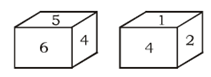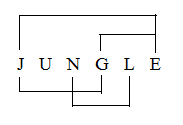# SSC CGL 2018 Practice Test Papers | Reasoning Ability (Day-33)

Dear Aspirants, Here we have given the Important SSC CGL Exam 2018 Practice Test Papers. Candidates those who are preparing for SSC CGL 2018 can practice these questions to get more confidence to Crack SSC CGL 2018 Examination.

[WpProQuiz 3211]

Click “Start Quiz” to attend these Questions and view Explanation

1) Select the related letters/ word/ number from the given alternatives.

Tripura: Agartala : : Mizoram : ?

a) Aizwal

b) Kohima

c) Shillong

d) Darjeeling

2) Select the related letters/ word/ number from the given alternatives.

Hacksaw : Metal : : Saw : ?

a) Wood

b) Gun

c) Nail

d) Plastic

3) Find the odd word/ letter/ number from the given responses.

a) 4556

b) 6684

c) 1450

d) 4806

4) A has less money than B but more than C. D has more money than A but less than B. E has more money than B. Who is the richest among them?

a) B

b) C

c) D

d) E

5) A has less money than B but more than C. D has more money than A but less than B. E has more money than B. Who have the least amount?

a) B

b) C

c) D

d) E

6) Which number will occur on the face opposite to 1?a) 6

b) 2

c) 3

d) 4

7) If 6+ 3 = 318, 13 + 7 = 691 and 10 + 9 = 190, then 7 + 2 =?

a) 514

b) 489

c) 984

c) 257

8) Which one set of letters when sequentially placed at the gaps in the given letter series shall complete it?

a _ c _ a b b _ a _ b c _ b c _ a b

a) cbcaaa

b) bcccab

c) bccaac

d) acbabc

9) Select the correct combination of mathematical signs to replace * signs and to balance the given equation?

35 * 7 * 5 * 1 * 5 * 245

a) × ÷ + – =

b) + × ÷ – =

c) – + ÷ × =

d) × + ÷ – =

10) How many pairs of letters are there in the word “JUNGLE” which has as many letters between them in the words as they have between them in the English alphabet?

a) One

b) Two

c) three

d) More than four

Agartala is capiytal of Tripura. Similarly Aizwal is the capital of Mizoram.

Metal is cut by Hacksaw. Similarly, Wood is cut by Saw.

4+5+5+6=20

6+6+8+4=24

2+4+5+0=11

4+8+0+6=18

The sum of all digits is even number except option c.

E > B > D > A > C

E > B > D > A > C

The face opposite to 1 is 6

6+3 = (6-3)|(6×3) = 318

Similarly,

7+2=(7-2)|(7×2)=514

ab c / c a b / b c a / a b c / a b c / c a b

35 × 7 + 5 ÷ 1 – 5 = 245

35 × 7 + 5 – 5 = 245

245 + 5 – 5 = 245

250 – 5 = 245